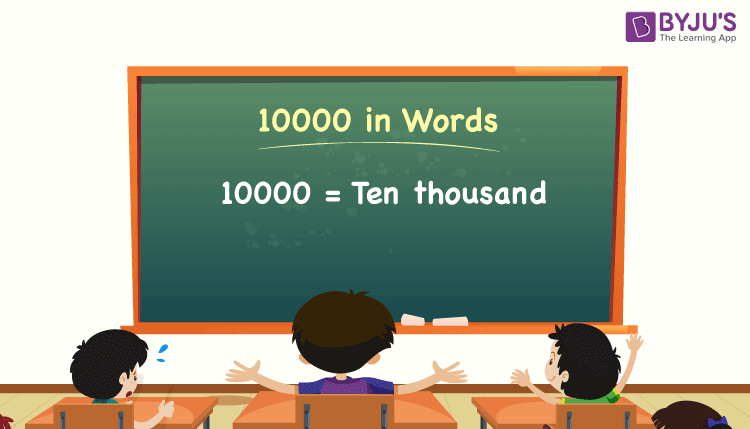# 10000 in Words

10000 in words can be written as Ten thousand. Suppose an EMI bill is generated in the first week of a month as Rs. 10,000, which can be read as “Ten thousand rupees”. As we know, 10000 is a cardinal number since it denotes an exact quantity.

 10000 in words: Ten thousand Ten thousand in numerical form: 10000

10000 in English words

We generally write numbers in words using the English alphabet. Thus, we can read 10000 in English words as “Ten thousand”.## How to Write 10000 in Words?

We can convert 10000 to words using a place value chart. This can be done as follows.

The number 10000 has 5 digits, so let’s make a chart that shows the place value up to 5 digits.

 Ten thousand Thousands Hundreds Tens Ones 1 0 0 0 0

Here, we can observe that, ones = 0, tens = 0, hundreds = 0, thousands = 0, Ten thousands = 1

Therefore, 10000 = 1 × Ten thousand = Ten thousand

Thus, we can write the expanded form as:

1 × Ten thousand + 0 × Thousand + 0 × Hundred + 0 × Ten + 0 × One

= 1 × 10000 + 0 × 1000 + 0 × 100 + 0 × 10 + 0 × 1

= 10000

= Ten thousand

Interesting way of writing 10000 in words:

1 = One

10 = Ten

100 = Hundred

1000 = Thousand

10000 = Ten thousand

10000 is a natural number that precedes 10001 and succeeds 9999.

10000 in words – Ten thousand

Is 10000 an odd number? – No

Is 10000 an even number? – Yes

Is 10000 a perfect square number? – Yes

What is the square root of 10000? – 100

Is 10000 a perfect cube number? – No

Is 10000 a prime number? – No

Is 10000 a composite number? – Yes

### Solved Example

Question: Write the value of 7600 + 2400 in words.

Solution:

Let us evaluate 7600 + 2400

7600 + 2400 = 10000 = Ten thousand

Therefore, the value of 7600 + 2400 in words is ten thousand.

## Frequently Asked Questions on 10000 in Words

Q1

### What is the correct spelling of 10000?

The spelling of 10000 in English words is Ten thousand, which means 10000 in words can be expressed as Ten thousand.

Q2

### How do you write 10000 rupees in words?

10000 rupees in words can be written as Ten thousand rupees.

Q3

### How many thousands will make 10000?

Ten thousands will be 10000, i.e. 10 × Thousand = 10 × 1000 = 10000 = Ten thousand.

Q4

### How do you write 10000 in the form of words on a cheque?

On a cheque, we generally write 10000 in words as Ten thousand rupees only.

Test your Knowledge on 10000 in Words